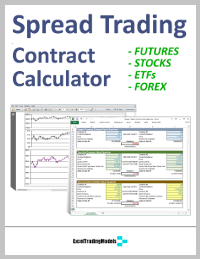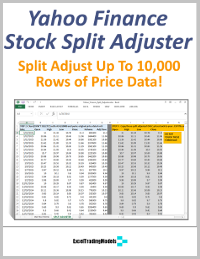Select Page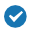### 3 detailed Excel Calculators PLUS User Guide### For Futures, Stocks, ETFs and Forex### 100% open Formulas to see it working### Save time with quick and flexible calculations### Contract Specifications, Tick Values and Unit Conversion sources provided### GUARANTEED 100% ACCURATE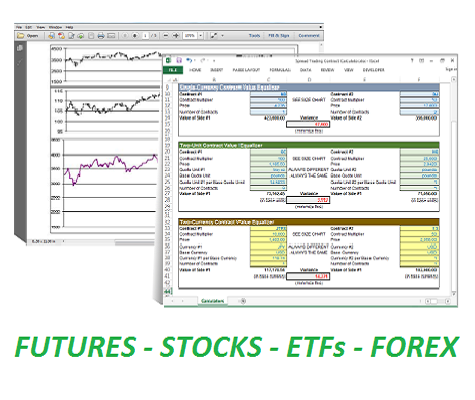When spread trading two different futures contracts, it is important to equalize the value of each side of the spread.

The short position in Contract #1 should be offset as closely as possible by a long position in Contract #2.

Constructing and maintaining a perfectly balanced spread can be difficult due to:

• Differences in the prices of the 2 assets (e.g. IBM stock at \$X/share and WMT stock at \$Y/share), and variations in their volatility and financial leverage.
• Differences in currency values of the 2 assets (e.g. Nikkei 225 futures denominated in \$USD and Yen)
• Differences in currency unit sizes of the 2 assets (e.g. dollars and cents, pounds and pence)
• Differences in the quote units in which the 2 assets are denominated (e.g. basis points and dollars)
• Differences in the contract multipliers of 2 different futures or options (e.g. full size S&P 500 futures vs. E-Mini S&P 500 futures)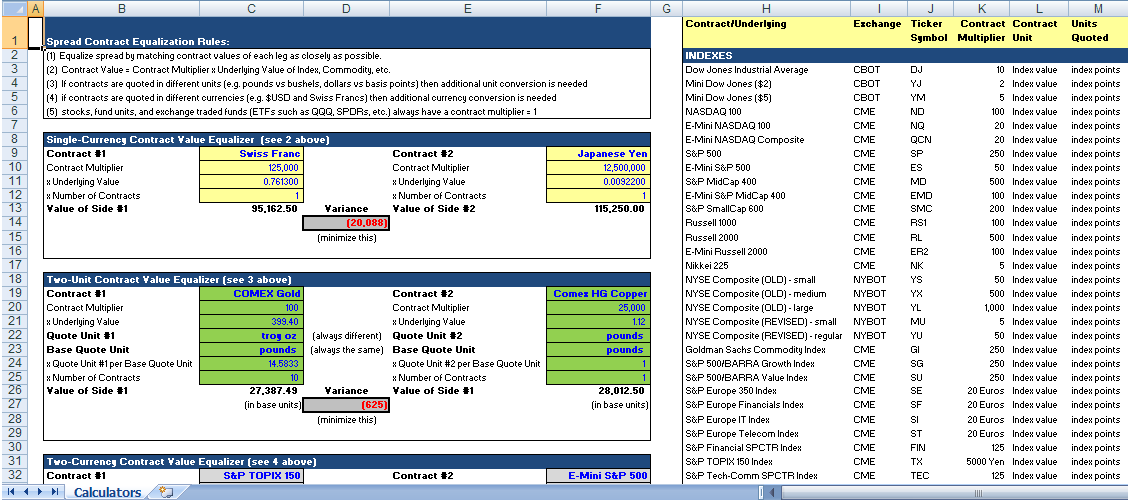The pre-built Excel Spread Calculator workbook lets you plug various contract parameters into 1 of 3 different Calculators, and balance the number of contracts to equalize the value of each spread leg.

For example:

• Two Futures Contracts denominated in a single currency but different units. COMEX USD Gold vs. Copper futures are denominated in 100 Troy Ounces and 25,000 Pounds, respectively. If Pounds are chosen as the base unit of spread measure, the Copper futures value must be converted into Troy Ounces, then the two contracts equalized by number of contracts. This apparently simple calculation entails 5 different inputs which the Spread Trading Contract Calculator handles correctly.
• Two futures contracts in different currencies. CME-listed Swiss Franc and Japanese Yen currency futures are denominated in CHF/USD and JPY/USD, respectively. In order to spread trade these two futures contracts, you must convert the denominator of the contracts into foreign-currency equivalent, thereby removing USD from the equation. The Spread Trading Contract Calculator does this easily.
• Two futures contracts denominated in different currencies and different units. If you would like to spread trade the S&P TOPIX 150 JPY equity index vs. the S&P 500 USD equity index, you need to make both a currency conversion and a unit conversion in the correct sequence, which takes 8 different inputs. This is done efficiently and accurately with the Spread Calculator in Excel.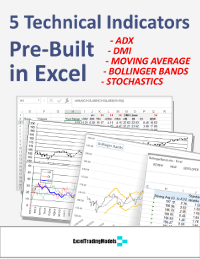# GET YOUR 5 FREE TECHNICAL INDICATORS

## Success! Your indicators are on their way!# FREE GETTING STARTED GUIDE

## Success! Your guide is on its way!